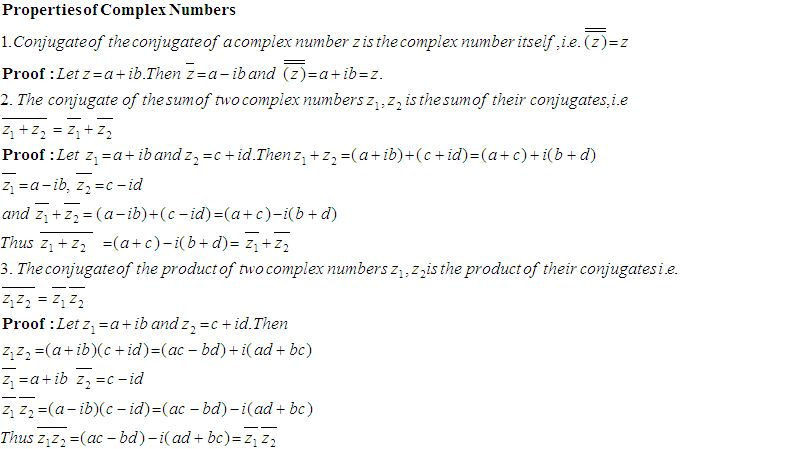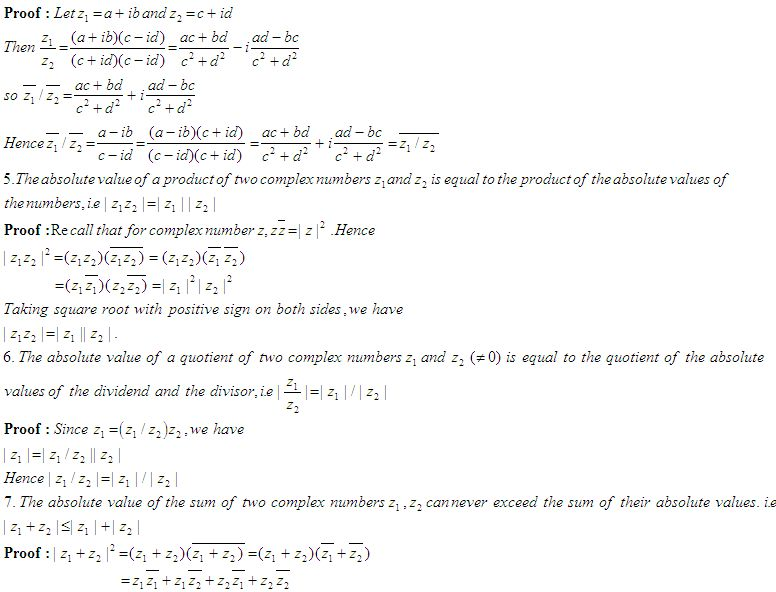Name: ___________________Date:___________________

 Email us to get an instant 20% discount on highly effective K-12 Math & English kwizNET Programs!

### High School Mathematics - 22.6 Some Properties of Complex NumbersDirections: Answer the following questions. Also write at least 5 examples of your own.
 Q 1: Conjugate of conjugate of 3i is ___.1/3i-3i3i Q 2: The conjugate of z1 + z2 given that z1 = 3+i5 and z2 = 6+i8 is ____.9+i13-9-13i9-i13 Q 3: If z = a+ib, what is the multiplicative inverse?a-ib1/a+ib1/a-ib Q 4: The conjugate of the product of two complex numbers z1 = 3+i7, z2 = 8+i535-i337-i5721-i42 Q 5: [(Ö2+iÖ3)+(Ö2-iÖ3)]/[(Ö3+iÖ2)+(Ö3-iÖ2)]Answer: Q 6: Find the conjugate of the number 49-i7.Answer: Q 7: Find x and y in 4x+i(3x-y) = 3-i6Answer: Q 8: Find x and y in (3y-2)+i(7-2x) = 0Answer: Question 9: This question is available to subscribers only! Question 10: This question is available to subscribers only!

#### Subscription to kwizNET Learning System costs less than \$1 per month & offers the following benefits:

• Unrestricted access to grade appropriate lessons, quizzes, & printable worksheets
• Instant scoring of online quizzes
• Progress tracking and award certificates to keep your student motivated
• Unlimited practice with auto-generated 'WIZ MATH' quizzes